### 3.7 Expressions

This section discusses particulars related to expressions appearing in Nektar++. Expressions in Nektar++ are used to describe spatially or temporally varying properties, for example

• velocity profiles on a boundary
• some reference functions (e.g. exact solutions)

which can be retrieved in the solver code.

Expressions appear as the content of `VALUE` attribute of

• parameter values;
• boundary condition type tags within `<REGION>` subsection of `<BOUNDARYCONDITIONS>`, e.g. `<D>`, `<N>` etc;
• expression declaration tag `<E>` within `<FUNCTION>` subsection.

The tags above declare expressions as well as link them to one of the field variables declared in `<EXPANSIONS>` section. For example, the declaration

1  <D VAR="u" VALUE="sin(PI*x)*cos(PI*y)" />

registers expression sin(πx)cos(πy) as a Dirichlet boundary constraint associated with field variable `u`.

Enforcing the same velocity profile at multiple boundary regions and/or field variables results in repeated re-declarations of a corresponding expression. Currently one cannot directly link a boundary condition declaration with an expression uniquely specified somewhere else, e.g. in the `<FUNCTION>` subsection. However this duplication does not affect an overall computational performance.

#### 3.7.1 Variables and coordinate systems

Declarations of expressions are formulated in terms of problem space-time coordinates. The library code makes a number of assumptions to variable names and their order of appearance in the declarations. This section describes these assumptions.

Internally, the library uses 3D global coordinate space regardless of problem dimension. Internal global coordinate system has natural basis (1,0,0),(0,1,0),(0,0,1) with coordinates `x`, `y` and `z`. In other words, variables `x`, `y` and `z` are considered to be first, second and third coordinates of a point (`x`, `y`, `z`).

Declarations of problem spatial variables do not exist in the current XML file format. Even though field variables are declarable as in the following code snippet,

1   <VARIABLES>
2     <V ID="0"> u </V>
3     <V ID="1"> v </V>
4   </VARIABLES>

there are no analogous tags for space variables. However an attribute `SPACE` of `<GEOMETRY>` section tag declares the dimension of problem space. For example,

1  <GEOMETRY DIM="1" SPACE="2"> ...
2  </GEOMETRY>

specifies 1D flow within 2D problem space. The number of spatial variables presented in expression declaration should match space dimension declared via `<GEOMETRY>` section tag.

The library assumes the problem space also has natural basis and spatial coordinates have names `x`, `y` and `z`.

Problem space is naturally embedded into the global coordinate space: each point of

• 1D problem space with coordinate x is represented by 3D point (x,0,0) in the global coordinate system;
• 2D problem space with coordinates (x,y) is represented by 3D point (x,y,0) in the global coordinate system;
• 3D problem space with coordinates (x,y,z) has the same coordinates in the global space coordinates.

Currently, there is no way to describe rotations and translations of problem space relative to the global coordinate system.

The list of variables allowed in expressions depends on the problem dimension:

• For 1D problems, expressions must make use of variable `x` only;
• For 2D problems, expressions should make use of variables `x` and `y` only;
• For 3D problems, expressions may use any of variables `x`, `y` and `z`.

Violation of these constraints yields unpredictable results of expression evaluation. The current implementation assigns magic value -9999 to each dimensionally excessive spacial variable appearing in expressions. For example, the following declaration

1  <GEOMETRY DIM="2" SPACE="2"> ...
2  </GEOMETRY> ...
3  <CONDITIONS> ...
4    <BOUNDARYCONDITIONS>
5       <REGION REF="0">
6         <D VAR="u" VALUE="x+y+z" /> <D VAR="v" VALUE="sin(PI*x)*cos(PI*y)" />
7       </REGION>
8     </BOUNDARYCONDITIONS>
9  ...
10  </CONDITIONS>

results in expression x + y + z being evaluated at spatial points (xi,yi,-9999) where xi and yi are the spacial coordinates of boundary degrees of freedom. However, the library behaviour under this constraint violation may change at later stages of development (e.g., magic constant 0 may be chosen) and should be considered unpredictable.

Another example of unpredictable behaviour corresponds to wrong ordering of variables:

1  <GEOMETRY DIM="1" SPACE="1"> ...
2  </GEOMETRY> ...
3  <CONDITIONS> ...
4    <BOUNDARYCONDITIONS>
5       <REGION REF="0">
6         <D VAR="u" VALUE="sin(y)" />
7       </REGION>
8     </BOUNDARYCONDITIONS>
9  ...
10  </CONDITIONS>

Here one declares 1D problem, so Nektar++ library assumes spacial variable is `x`. At the same time, an expression sin(y) is perfectly valid on its own, but since it does not depend on `x`, it will be evaluated to constant sin(-9999) regardless of degree of freedom under consideration.

##### 3.7.1.1 Time dependence

Variable `t` represents time dependence within expressions. The boundary condition declarations need to add an additional property `USERDEFINEDTYPE="TimeDependent"` in order to flag time dependency to the library.

##### 3.7.1.2 Syntax of expressions

Analytic expressions are formed of

• brackets (). Bracketing structure must be balanced.
• real numbers: every representation is allowed that is correct for `boost::lexical_cast<double>()`, e.g.
1   1.2, 1.2e-5, .02
• mathematical constants

 Identifier Meaning Real Value Fundamental constants E Natural Logarithm 2.71828182845904523536 PI π 3.14159265358979323846 GAMMA Euler Gamma 0.57721566490153286060 DEG deg/radian 57.2957795130823208768 PHI golden ratio 1.61803398874989484820 Derived constants LOG2E log 2e 1.44269504088896340740 LOG10E log 10e 0.43429448190325182765 LN2 log e2 0.69314718055994530942 PI_2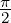1.57079632679489661923 PI_4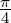0.78539816339744830962 1_PI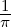0.31830988618379067154 2_PI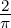0.63661977236758134308 2_SQRTPI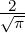1.12837916709551257390 SQRT2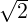1.41421356237309504880 SQRT1_2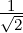0.70710678118654752440
• parameters: alphanumeric names with underscores, e.g. `GAMMA_123`, `GaM123_45a_`, `_gamma123` are perfectly acceptable parameter names. However parameter name cannot start with a numeral. Parameters must be defined with `<PARAMETERS>...</PARAMETERS>`. Parameters play the role of constants that may change their values in between of expression evaluations.
• variables (i.e., `x, y, z` and `t`)
• unary minus operator (e.g. `-x`)
• binary arithmetic operators `+, -, *, /, ^` Powering operator allows using real exponents (it is implemented with `std::pow()` function)
• boolean comparison operations `<, <=, >, >=, ==` evaluate their sub-expressions to real values 0.0 or 1.0.
• mathematical functions of one or two arguments:

 Identifier Meaning `abs(x)` absolute value |x| `asin(x)` inverse sine arcsin x `acos(x)` inverse cosine arccosx `ang(x,y)` computes polar coordinate θ = arctan(y∕x) from (x,y) `atan(x)` inverse tangent arctan x `atan2(y,x)` inverse tangent function (used in polar transformations) `ceil(x)` round up to nearest integer ⌈x⌉ `cos(x)` cosine cosx `cosh(x)` hyperbolic cosine cosh x `exp(x)` exponential ex `fabs(x)` absolute value (equivalent to `abs`) `floor(x)` rounding down ⌊x⌋ `log(x)` logarithm base e, ln x = log x `log10(x)` logarithm base 10, log 10x `rad(x,y)` computes polar coordinate r =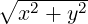from (x,y) `sin(x)` sine sin x `sinh(x)` hyperbolic sine sinh x `sqrt(x)` square root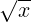`tan(x)` tangent tan x `tanh(x)` hyperbolic tangent tanh x

These functions are implemented by means of the cmath library: http://www.cplusplus.com/reference/clibrary/cmath/. Underlying data type is `double` at each stage of expression evaluation. As consequence, complex-valued expressions (e.g. (-2)0.123) get value `nan` (not a number). The operator `^` is implemented via call to `std::pow()` function and accepts arbitrary real exponents.

• random noise generation functions. Currently implemented is `awgn(sigma)` - Gaussian Noise generator, where σ is the variance of normal distribution with zero mean. Implemented using the `boost::mt19937` random number generator with boost variate generators (see http://www.boost.org/libs/random)

##### 3.7.1.3 Examples

Some straightforward examples include

• Basic arithmetic operators: `0.5*0.3164/(3000^0.25)`
• Simple polynomial functions: `y*(1-y)`
• Use of values defined in `PARAMETERS` section: `-2*Kinvis*(x-1)`
• More complex expressions involving trigonometric functions, parameters and constants: `(LAMBDA/2/PI)*exp(LAMBDA*x)*sin(2*PI*y)`
• Boolean operators for multi-domain functions: `(y<0)*sin(y) + (y>=0)*y`

#### 3.7.2 Performance considerations

Processing expressions is split into two stages:

• parsing with pre-evaluation of constant sub-expressions,
• evaluation to a number.

Parsing of expressions with their partial evaluation take place at the time of setting the run up (reading an XML file). Each expression, after being pre-processed, is stored internally and quickly retrieved when it turns to evaluation at given spatial-time point(s). This allows to perform evaluation of expressions at a large number of spacial points with minimal setup costs.

##### 3.7.2.1 Pre-evaluation details

Partial evaluation of all constant sub-expressions makes no sense in using derived constants from table above. This means, either make use of pre-defined constant `LN10^2` or straightforward expression `log10(2)^2` results in constant `5.3018981104783980105` being stored internally after pre-processing. The rules of pre-evaluation are as follows:

• constants, numbers and their combinations with arithmetic, analytic and comparison operators are pre-evaluated,
• appearance of a variable or parameter at any recursion level stops pre-evaluation of all upper level operations (but doesn’t stop pre-evaluation of independent parallel sub-expressions).

For example, declaration

1     <D VAR="u" VALUE="exp(-x*sin(PI*(sqrt(2)+sqrt(3))/2)*y )" />

results in expression `exp(-x*(-0.97372300937516503167)*y )` being stored internally: sub-expression `sin(PI*(sqrt(2)+sqrt(3))/2)` is evaluated to constant but appearance of `x` and `y` variables stops further pre-evaluation.

Grouping predefined constants and numbers together helps. Its useful to put brackets to be sure your constants do not run out and become factors of some variables or parameters.

Expression evaluator does not do any clever simplifications of input expressions, which is clear from example above (there is no point in double negation). The following subsection addresses the simplification strategy.

##### 3.7.2.2 Preparing expression

The total evaluation cost depends on the overall number of operations. Since evaluator is not making simplifications, it worth trying to minimise the total number of operations in input expressions manually.

Some operations are more computationally expensive than others. In an order of increasing complexity:

• `+, -, <, >, <=, >=, ==, `
• `*, /, abs, fabs, ceil, floor,`
• ```^, sqrt, exp, log, log10, sin, cos, tan, sinh, cosh, tanh, asin, acos, atan```.

For example,

• `x*x` is faster than `x^2` — it is one double multiplication vs generic calculation of arbitrary power with real exponents.
• `(x+sin(y))^2` is faster than `(x+sin(y))*(x+sin(y))` - sine is an expensive operation. It is cheaper to square complicated expression rather than compute it twice and add one multiplication.
• An expression ```exp(-41*( (x+(0.3*cos(2*PI*t)))^2 + (0.3*sin(2*PI*t))^2 ))``` makes use of 5 expensive operations (`exp`, `sin`, `cos` and power `^` twice) while an equivalent expression `exp(-41*( x*x+0.6*x*cos(2*PI*t) + 0.09 ))` uses only 2 expensive operations.

If any simplifying identity applies to input expression, it may worth applying it, provided it minimises the complexity of evaluation. Computer algebra systems may help.

##### 3.7.2.3 Vectorized evaluation

Expression evaluator is able to calculate an expression for either given point (its space-time coordinates) or given array of points (arrays of their space-time coordinates, it uses SoA). Vectorized evaluation is faster then sequential due to a better data access pattern. Some expressions give measurable speedup factor 4.6. Therefore, if you are creating your own solver, it worth making vectorized calls.